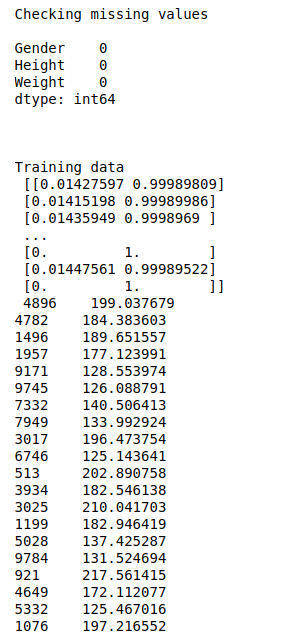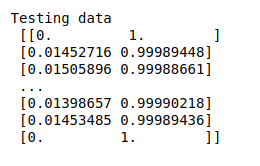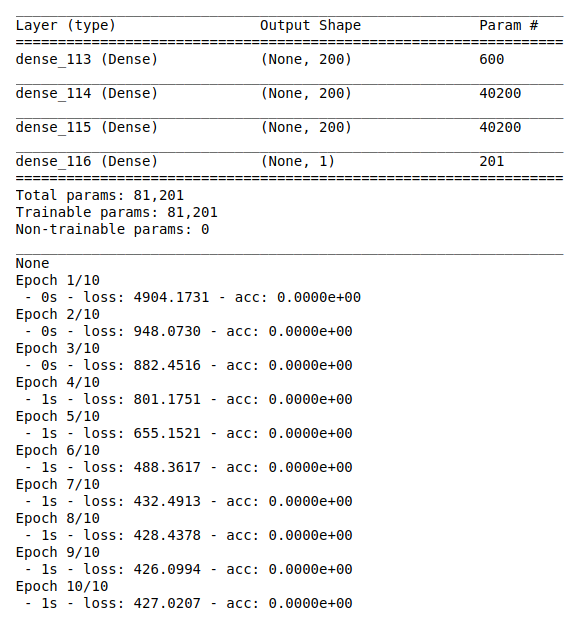• #5, First Floor, 4th Street Dr. Subbarayan Nagar Kodambakkam, Chennai-600 024 Landmark : Samiyar Madam
• pro@slogix.in
• +91- 81240 01111

### How to build regression model using keras for predicting students weights in python?

###### Description

To write a piece of python code to find, search and split particular string in a file using regular expressions.

###### Input

Height-Weight data set. (Kaggle)

R-squared

###### Process

Import keras library.

Import other needed libraries.

Fix the independent and dependent variable.

Normalize the independent variable.

Split the data into train and test data.

Build the DNN model.

Compile the DNN model with appropriate loss and activation functions.

Fit the train data to the model.

Predict the test data.

Check results for new instance.

Calculate r-squared value.

###### Sample Code

#import necessary libraries
import warnings
warnings.filterwarnings(“ignore”)
import pandas as pd
import numpy as np
import matplotlib.pyplot as plt
import seaborn as sns
from keras.models import Sequential
from keras.layers import Dense
from sklearn.model_selection import train_test_split
from sklearn.preprocessing import Normalizer

#Make it as a data frame
df = pd.DataFrame(data)

#Checking missing values
print(“Checking missing values\n”)
print(df.isnull().sum())

#Pre-processing
from sklearn import preprocessing

# label_encoder object knows how to understand word labels.
label_encoder = preprocessing.LabelEncoder()

# Encode labels in column ‘Gender’.
df[‘Gender’]= label_encoder.fit_transform(df[‘Gender’])

#feature selection
X = df.iloc[:,0:2]
y = df.iloc[:,2]

#normalize the data
transformer = Normalizer().fit(X)
X = transformer.transform(X)
print(“\n\n”)

#Split the data into train and testing
X_train, X_test, Y_train, Y_test = train_test_split(X, y, test_size=0.1, random_state=42)

#Print training data
print(“Training data\n”,X_train,”\n”,Y_train)
print(“\n\n”)

#Print testing data
print(“Testing data\n”,X_test)
print(“\n\n”)

#create model
model = Sequential()
shape = X_train.shape

print(model.summary())

#Compile the model

#Here we train the Network.
model.fit(X_train, Y_train, batch_size=30, epochs = 10, verbose = 2)

#Here we evaluate the model
score,acc = model.evaluate(X_test,Y_test,verbose = 2,batch_size = 30)

#predict test data
y_pred = model.predict(X_test)

#predict new value
print(“\n”)
new = [[1,69.88179586]]
transformer_new = Normalizer().fit(new)
new = transformer_new.transform(new)
new_pred = model.predict(new)
print(“New prediction\n”,new_pred,”\n”)

#r squared
from sklearn.metrics import r2_score
R_squared = r2_score(Y_test,y_pred)
print(“R-squared\n”,round(R_squared))

###### Screenshots